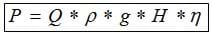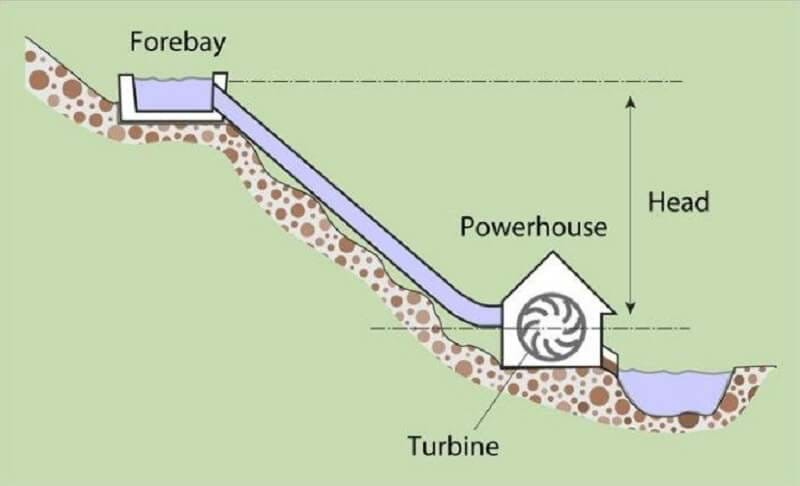# Online Hydro Power Calculator

#### How to Calculate Hydro Electrical Energy

We have got multiple calculators that will allow you to calculate the hydroelectrical power that could get generated.
From micro-hydro turbines and traditional sizes to run of river and tidal power stations, we have a calculator that will allow you to see its approximate power generation abilities.Let's break down what each of the letters represents in the Hydroelectric Power Calculation(if you are just looking to use our calculator keep scrolling down). Remember this calculation is only the theoretical power that should be available from falling water.

## Hydro Power Calculation Formula

P = Q * ρ * g * H * η

P = the electric power produced in kVA
Q = flow rate in the pipe (m3/s)
ρ = density (kg/m3), Water = 1000
g = 9.81 = Acceleration of gravity (m/s²)
H = waterfall height (m)
η = global efficiency ratio (usually between 0.7 and 0.9)
If you are using a micro Microhydro power System an efficiency of 53% so you need to use .53 for η,

## Hydro Power Calculator

##### P = Q * ρ * g * H * η

Remember if you are using this for micro hydropower to use a lower efficiency around 50%

A low head site has a head of below 10 metres. In this case you need to have a good volume of water flow if you are to generate much electricity. A high head site has a head of above 20 metres. In this case you can get away with not having a large flow of water, because gravity will give what you have an energy boost.

Calculation of Hydro Power www.reuk.co.uk/

## How to determine your (Q) Flow Rate

Q = A * v

A = Where A is the Cross-sectional area in m² (meters squared)
v = Is the Flow velocity in m/s (meters per second)

## How to determine Run Of River Power Generation

P = 0.5 * η * ρ * Q * v²

When you are using a ROR system, the formula to calculate the expected power creation is slightly different. These stations will use the kinetic energy of the flow and gravity, and the head does not get used anymore.

## How to Determine the Head/Height

Head refers to the vertical height that the water will drop down by. The head gets measured in meters or feet.Remember to keep power generation at its highest level. It will help if you keep making sure that the inlet screen is clear of any debris. Removing any built-up debris ensures your hydroelectric system maintains the maximum system head.

### Don't forget to Check for Rebates & Incentives

Remember many states offer financial incentives for people who install different types of alternative energy systems.